|

# 达宝利揭真相:德国人长寿的十大秘诀

2016年5月，世界卫生组织发布了《2016年世界卫生统计》报告显示：全球人均寿命为71.4岁，中国人均寿命76.1岁，德国人均寿命81岁。德国为何在短短百余年间成为全球著名的长寿国度之一？这是和德国人的传统习惯密不可分的，小编带您领略下德国人长寿的十大秘诀。

【秘诀1】每周五都会吃鱼

德国人到星期五，无论公司食堂还是大中小学食堂，无论饭店餐馆还是寻常百姓家，主菜都是鱼。为什么德国人星期五都爱吃鱼呢？

这是因为它的宗教渊源。在西方，鱼是不属于肉类的，星期五不吃肉，可以吃鱼。德国人意识到吃太多红肉对身体健康不利，所以现在越来越多的德国人愿意吃鱼，正好借星期五“强制”吃鱼，成为一种全民饮食习惯。

鱼类蛋白富含蛋氨酸和牛磺酸，有助于降低高血压和脑卒中的发病率，而鱼油富含不饱和脂肪酸，也有降压作用。另外研究发现，吃鱼还可降低结直肠癌的发病率。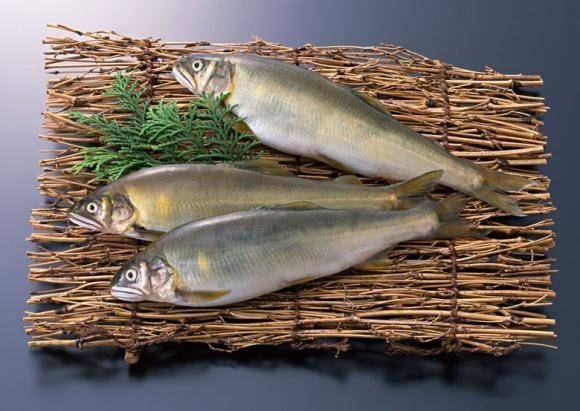【秘诀2】从小喝牛奶，连做菜也要放牛奶

事实上，没有一个国家的人们像德国人那么爱喝牛奶。德国人每年要喝掉约85公斤的牛奶，堪称世界之最。他们从小就喝下大量牛奶，连做菜也放牛奶，类似我们中国的勾芡，所以德国人似乎都不缺钙。

牛奶营养价值很高，除含有蛋白质外，还含有脂肪、糖、钙、磷等许多营养成分。一般来说，成人一天喝300～500毫升牛奶为宜。德国人早餐种类不多，但保持了最简单的营养搭配——牛奶加蜂蜜。简单的阳光早餐，赋予德国人一天好精神。【秘诀3】每天“站比坐多”

《国家癌症研究》杂志上的科研成果表明，久坐的人同坐下时间最短者相比，患结肠癌、子宫内膜癌以及肺癌等疾病的风险要更高。德国人就不怎么喜欢“坐”。

德国人喜欢站着办公：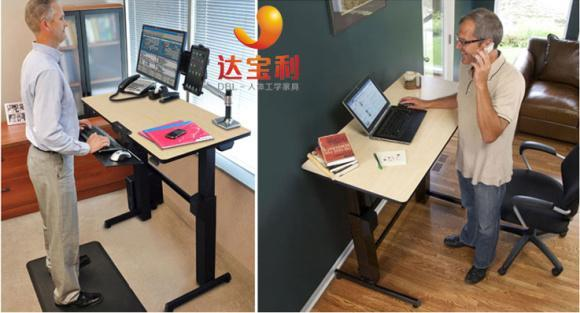在德国，一些备受腰椎病困扰的办公族，开始摆脱久坐、尝试“站立式办公”。这样有利于缓解腰椎不适的症状。

德国人喜欢站着开会：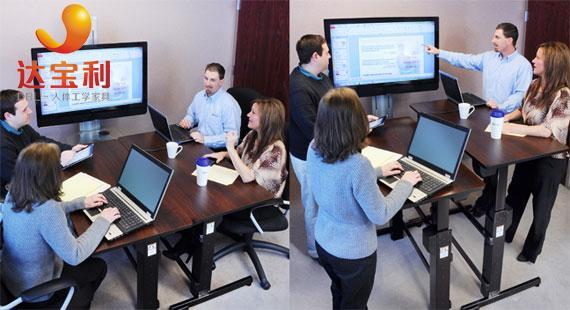法兰克福的一些企业开会前，会拿出一只小闹钟并将闹铃时间定为20分钟，职员们就站在原地热烈地交换意见，闹钟一响就结束会议。不说废话，仅简略地谈论要点，所以事半功倍。

德国学生喜欢站着上课：德国有的中学的孩子们想站着或者坐着听课都可以。他们的学习桌是可以升降并且可以移动的。老师们认为，这种不固定位置、不固定姿势的上课方式提高了孩子们的学习兴趣，对肥胖的孩子还能起到减肥的作用。

达宝利人体工学家具是国内领先的站式办公家具升降办公桌的专业销售商，在北上广深津杭六座城市设立八家直营店铺，在天猫、京东等主流电商平台均设有旗舰店。

【秘诀4】古代骑马，今日骑马式坐姿

德国人在古代时就喜欢骑马，现在的德国人直接把骑马的姿势引入日常坐姿之中，经常使用“马鞍椅”。德国人发现模仿似坐非坐，似站非站的骑马的坐姿，有点中国武术的锻炼姿势“蹲马步”的痕迹。半站的姿势使得马鞍椅不需要靠背，上半身的重量主要由坐骨承担，而非肌肉等软组织，所以上身躯干可以自然保持挺直的状态，不会产生坐传统座椅时发生的弯腰驼背现象，达到缓解腰背疼痛、减少腹部脂肪堆积、维持头脑清醒、保护生殖系统、改善血液循环的目的。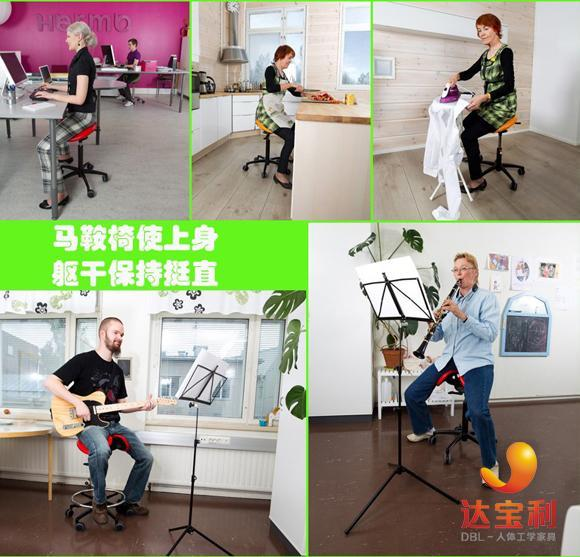马鞍椅和骑马式办公姿势从人体工学角度来讲更适合久坐和操作电脑，将逐步替代传统的座椅和坐姿。达宝利人机工学家具是欧洲马鞍椅在中国地区的战略合作伙伴，您可至天猫、京东等达宝利电商平台咨询或至分布于北上广深津杭六城八店进行现场体验。

【秘诀5】散步是德国人的传统爱好

德国是世界啤酒之乡，但这里的“啤酒肚”却逐年减少。大约2/3的德国人每周至少参加两次体育锻炼，大部分成年人每天至少散步15分钟。尤其是每个周日，更是他们的“散步日”。

散步不但能缓解工作的紧张和精神的疲乏，还能促使血管弹性的增加，特别是腿的持续运动，可促使更多的血液回到心脏，有利于改善血液循环，提高心脏的工作效率。

美国心脏病协会发布的研究称，散步是减轻体重、保护心脏正常功能和燃烧热量的最佳锻炼方式。每天散步30分钟就可以大大降低罹患多种疾病的风险。比如，与久坐不动者相比，经常散步的人因结肠癌死亡危险更低。【秘诀6】不比吃穿，自我放松

德国人自我放松的心态是健康的基石。

德国人平日的吃饭、穿衣戴帽都很随意，不会攀比，也不会去贷款买房子，虽然并不是买不起。其实，德国的工作压力也很大，但他们常对自己的生活满意，不想背负沉重的贷款负担，很多人干脆一生都租房子住。

在德国人眼中，房子对家、对幸福感没有直接的影响。房子以它最原始和最基本的形态和意义出现在他们的生活中。

积极心态获得的两大报酬，就是心理健康和身体健康。从某种意义上说，心态决定健康。当人的精神状态乐观欢快时，机体内的多种生理过程变得活跃，此时，防御功能也发挥得比较充分。当免疫功能增强时，自然就不容易受到疾病的侵犯。【秘诀7】多动脑勤动手，收获乐趣和成就感

另世界惊叹的德国制造技术来自德国人多动脑勤动手的传统习惯，当世界都在推行以理论研究为核心的本科教育的时候，德国人仍在坚持职业教育和专科教育，为德国的制造业输送着一代又一代的合格的产业工人。

德国人不仅爱动脑更爱动手，所以房屋的楼梯坏了，水管漏了，割草机失灵了，德国人一般不会找人去修理，一个是因为德国的人工很贵，另一个是因为德国男人家中几乎都配有全套的维修工具，而且他们也乐于动手去维修甚至制造一些东西，比如给孩子的秋千、自制的家具等等。例如环保无甲醛的需要人们动脑动手去拼装的积木式家具在德国就很受欢迎。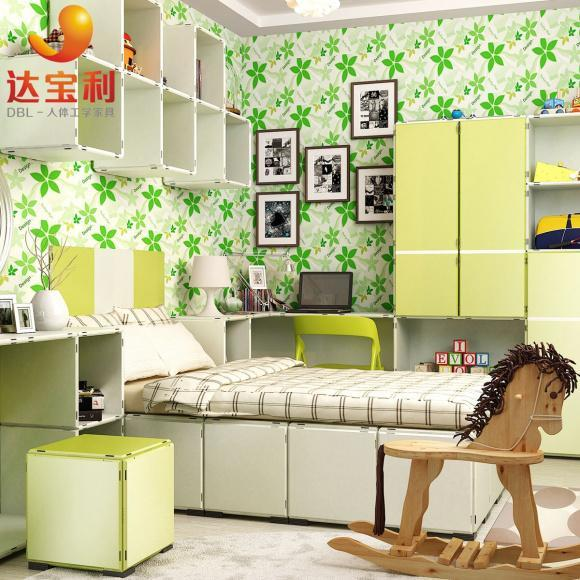积木式家具又称之为模块化家具，是国际流行的新材料家具，是指消费者像搭建积木一样将不同功能、规格及颜色的基本家具模块按照自己的实际需求和创意自由进行组装、调整样式，满足不同空间不同功能个性化要求的家具。达宝利积木式家具的每片材料都是环保树脂粒加工成型，不含甲醛，防水耐脏，拆装方面，承重可达255公斤/块，因此可被组装成走台、床铺、书桌、椅凳等高承重家具，受到各国消费者的喜爱。

【秘诀8】懂休闲，休息日回归自然

德国人的工作态度一丝不苟，但他们也相信持续工作不休息，工作效率必定变差，因此德国人非常重视休闲生活。

对德国人而言，休息是神圣不可侵犯的领域，周末时间绝对要留给自己。很少有人会在周末工作，更不可能利用周末去应付客户。即使再热衷工作的人，也深信唯有充分休息才能彻底充电、恢复活力。

德国人周末喜欢去户外，重回大自然，没有远足条件的，也去当地公园、植物园等“绿色地带”，有助于提升情绪和自信心。经常接触自然界的德国人更少生气、抑郁和紧张。户外散步和骑自行车等低强度有氧运动可降低心脏病风险。快走、钓鱼、划船和种花种菜等都是很好的户外运动方式。

健康可以攒，疲劳可不行！我们常说的疲劳主要是生理疲劳和心理疲劳，长期紧张工作，这种双重疲劳的压迫会带来很大的健康隐患。积攒疲劳容易导致生理功能、免疫功能、神经调节功能紊乱，最常见的结果是感冒，最恐怖的是癌症，最危急的是心脑血管疾病。

德国人不仅重视自己的休息时间，也非常注重他人的休息时间。如果没有急事，他们平日的晚上七点钟以后和周末、假日，都不会打电话到别人家。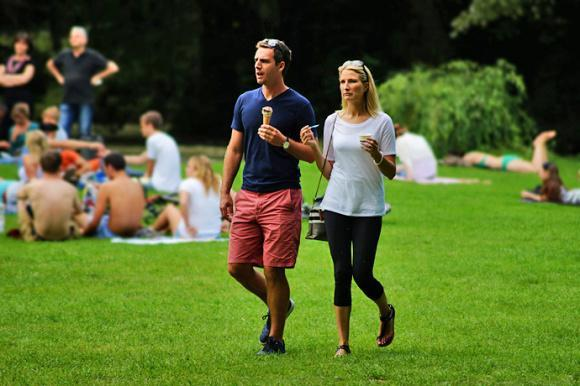【秘诀9】不服老，退休也会安排生活

学会如何安排自己的独处时间并不是一件简单的事情。中国的很多年青人就缺乏此方面的训练，一旦休息就是宅在家里熬夜赖床、玩电脑、看电影、打游戏，甚至不想洗漱、做饭，这样的时间安排反而让他们更加疲惫。

再看中国很多退休的老人出现了退休综合征：要么在家看电视，要么坐在墙根下发呆，要么和邻居亲友打麻将，每天看似很忙，内心却得不到充实。每天觉得手脚都没地放，身体健康状况也有所下降，有的会烦躁，有的会低落……看什么都不大顺眼了，严重的还会出现抑郁、焦虑、身体不适等。

德国的年轻人喜欢进行户外运动，参加聚会，合理安排个人生活。而德国的老人们则不服老，许多人主动延后退休。在德国街头，一些老年人专门为外国人指路，为盲人读报，就这样，他们找到了乐子，生活由此而充实幸福。

退休是另一种新生活的起点，可以自由安排时间，做自己想做的事。你可以重拾年轻时未完成的梦想，可以用画笔、相机、打字，把自己的生活展现出来，享受退休后的“第二青春”。【秘诀10】拒绝非必要性药物，吃药严格遵医嘱

在吃药上，我们真该好好学学德国人。中国人现在肾脏疾病增多的原因之一，就是自己滥用药。而德国人从来不随意买药吃，即便服药也严格地遵照医生指导。“是药三分毒”在德国也适用，他们只会在不得不用药时才用，错用、滥用药物都是非常危险的。

当我们毫不自知地吃保健品、非合理地滥用药物时，无形间可能就会带来肝损伤、肾损伤。一些疾病也是可以通过食疗方法解决的，这样避免了大量药物中有毒有害物质，身体之中的杂质变少了，寿命自然就会得到延长，因此在生活中拒绝非必要性的药物和保健品对于长寿来说是很重要的一件事情。`声明：本文由入驻焦点开放平台的作者撰写，除焦点官方账号外，观点仅代表作者本人，不代表焦点立场错误信息举报电话： 400-099-0099，邮箱：jubao@vip.sohu.com，或点此进行意见反馈，或点此进行举报投诉。`A B C D E F G H J K L M N P Q R S T W X Y Z
A - B - C - D - E
• A
• 鞍山
• 安庆
• 安阳
• 安顺
• 安康
• 澳门
• B
• 北京
• 保定
• 包头
• 巴彦淖尔
• 本溪
• 蚌埠
• 亳州
• 滨州
• 北海
• 百色
• 巴中
• 毕节
• 保山
• 宝鸡
• 白银
• 巴州
• C
• 承德
• 沧州
• 长治
• 赤峰
• 朝阳
• 长春
• 常州
• 滁州
• 池州
• 长沙
• 常德
• 郴州
• 潮州
• 崇左
• 重庆
• 成都
• 楚雄
• 昌都
• 慈溪
• 常熟
• D
• 大同
• 大连
• 丹东
• 大庆
• 东营
• 德州
• 东莞
• 德阳
• 达州
• 大理
• 德宏
• 定西
• 儋州
• 东平
• E
• 鄂尔多斯
• 鄂州
• 恩施
F - G - H - I - J
• F
• 抚顺
• 阜新
• 阜阳
• 福州
• 抚州
• 佛山
• 防城港
• G
• 赣州
• 广州
• 桂林
• 贵港
• 广元
• 广安
• 贵阳
• 固原
• H
• 邯郸
• 衡水
• 呼和浩特
• 呼伦贝尔
• 葫芦岛
• 哈尔滨
• 黑河
• 淮安
• 杭州
• 湖州
• 合肥
• 淮南
• 淮北
• 黄山
• 菏泽
• 鹤壁
• 黄石
• 黄冈
• 衡阳
• 怀化
• 惠州
• 河源
• 贺州
• 河池
• 海口
• 红河
• 汉中
• 海东
• 怀来
• I
• J
• 晋中
• 锦州
• 吉林
• 鸡西
• 佳木斯
• 嘉兴
• 金华
• 景德镇
• 九江
• 吉安
• 济南
• 济宁
• 焦作
• 荆门
• 荆州
• 江门
• 揭阳
• 金昌
• 酒泉
• 嘉峪关
K - L - M - N - P
• K
• 开封
• 昆明
• 昆山
• L
• 廊坊
• 临汾
• 辽阳
• 连云港
• 丽水
• 六安
• 龙岩
• 莱芜
• 临沂
• 聊城
• 洛阳
• 漯河
• 娄底
• 柳州
• 来宾
• 泸州
• 乐山
• 六盘水
• 丽江
• 临沧
• 拉萨
• 林芝
• 兰州
• 陇南
• M
• 牡丹江
• 马鞍山
• 茂名
• 梅州
• 绵阳
• 眉山
• N
• 南京
• 南通
• 宁波
• 南平
• 宁德
• 南昌
• 南阳
• 南宁
• 内江
• 南充
• P
• 盘锦
• 莆田
• 平顶山
• 濮阳
• 攀枝花
• 普洱
• 平凉
Q - R - S - T - W
• Q
• 秦皇岛
• 齐齐哈尔
• 衢州
• 泉州
• 青岛
• 清远
• 钦州
• 黔南
• 曲靖
• 庆阳
• R
• 日照
• 日喀则
• S
• 石家庄
• 沈阳
• 双鸭山
• 绥化
• 上海
• 苏州
• 宿迁
• 绍兴
• 宿州
• 三明
• 上饶
• 三门峡
• 商丘
• 十堰
• 随州
• 邵阳
• 韶关
• 深圳
• 汕头
• 汕尾
• 三亚
• 三沙
• 遂宁
• 山南
• 商洛
• 石嘴山
• T
• 天津
• 唐山
• 太原
• 通辽
• 铁岭
• 泰州
• 台州
• 铜陵
• 泰安
• 铜仁
• 铜川
• 天水
• 天门
• W
• 乌海
• 乌兰察布
• 无锡
• 温州
• 芜湖
• 潍坊
• 威海
• 武汉
• 梧州
• 渭南
• 武威
• 吴忠
• 乌鲁木齐
X - Y - Z
• X
• 邢台
• 徐州
• 宣城
• 厦门
• 新乡
• 许昌
• 信阳
• 襄阳
• 孝感
• 咸宁
• 湘潭
• 湘西
• 西双版纳
• 西安
• 咸阳
• 西宁
• 仙桃
• 西昌
• Y
• 运城
• 营口
• 盐城
• 扬州
• 鹰潭
• 宜春
• 烟台
• 宜昌
• 岳阳
• 益阳
• 永州
• 阳江
• 云浮
• 玉林
• 宜宾
• 雅安
• 玉溪
• 延安
• 榆林
• 银川
• Z
• 张家口
• 镇江
• 舟山
• 漳州
• 淄博
• 枣庄
• 郑州
• 周口
• 驻马店
• 株洲
• 张家界
• 珠海
• 湛江
• 肇庆
• 中山
• 自贡
• 资阳
• 遵义
• 昭通
• 张掖
• 中卫

1室1厅1厨1卫1阳台

1
2
3
4
5

0
1
2

1

1

0
1
2
3报名成功，资料已提交审核A B C D E F G H J K L M N P Q R S T W X Y Z
A - B - C - D - E
• A
• 鞍山
• 安庆
• 安阳
• 安顺
• 安康
• 澳门
• B
• 北京
• 保定
• 包头
• 巴彦淖尔
• 本溪
• 蚌埠
• 亳州
• 滨州
• 北海
• 百色
• 巴中
• 毕节
• 保山
• 宝鸡
• 白银
• 巴州
• C
• 承德
• 沧州
• 长治
• 赤峰
• 朝阳
• 长春
• 常州
• 滁州
• 池州
• 长沙
• 常德
• 郴州
• 潮州
• 崇左
• 重庆
• 成都
• 楚雄
• 昌都
• 慈溪
• 常熟
• D
• 大同
• 大连
• 丹东
• 大庆
• 东营
• 德州
• 东莞
• 德阳
• 达州
• 大理
• 德宏
• 定西
• 儋州
• 东平
• E
• 鄂尔多斯
• 鄂州
• 恩施
F - G - H - I - J
• F
• 抚顺
• 阜新
• 阜阳
• 福州
• 抚州
• 佛山
• 防城港
• G
• 赣州
• 广州
• 桂林
• 贵港
• 广元
• 广安
• 贵阳
• 固原
• H
• 邯郸
• 衡水
• 呼和浩特
• 呼伦贝尔
• 葫芦岛
• 哈尔滨
• 黑河
• 淮安
• 杭州
• 湖州
• 合肥
• 淮南
• 淮北
• 黄山
• 菏泽
• 鹤壁
• 黄石
• 黄冈
• 衡阳
• 怀化
• 惠州
• 河源
• 贺州
• 河池
• 海口
• 红河
• 汉中
• 海东
• 怀来
• I
• J
• 晋中
• 锦州
• 吉林
• 鸡西
• 佳木斯
• 嘉兴
• 金华
• 景德镇
• 九江
• 吉安
• 济南
• 济宁
• 焦作
• 荆门
• 荆州
• 江门
• 揭阳
• 金昌
• 酒泉
• 嘉峪关
K - L - M - N - P
• K
• 开封
• 昆明
• 昆山
• L
• 廊坊
• 临汾
• 辽阳
• 连云港
• 丽水
• 六安
• 龙岩
• 莱芜
• 临沂
• 聊城
• 洛阳
• 漯河
• 娄底
• 柳州
• 来宾
• 泸州
• 乐山
• 六盘水
• 丽江
• 临沧
• 拉萨
• 林芝
• 兰州
• 陇南
• M
• 牡丹江
• 马鞍山
• 茂名
• 梅州
• 绵阳
• 眉山
• N
• 南京
• 南通
• 宁波
• 南平
• 宁德
• 南昌
• 南阳
• 南宁
• 内江
• 南充
• P
• 盘锦
• 莆田
• 平顶山
• 濮阳
• 攀枝花
• 普洱
• 平凉
Q - R - S - T - W
• Q
• 秦皇岛
• 齐齐哈尔
• 衢州
• 泉州
• 青岛
• 清远
• 钦州
• 黔南
• 曲靖
• 庆阳
• R
• 日照
• 日喀则
• S
• 石家庄
• 沈阳
• 双鸭山
• 绥化
• 上海
• 苏州
• 宿迁
• 绍兴
• 宿州
• 三明
• 上饶
• 三门峡
• 商丘
• 十堰
• 随州
• 邵阳
• 韶关
• 深圳
• 汕头
• 汕尾
• 三亚
• 三沙
• 遂宁
• 山南
• 商洛
• 石嘴山
• T
• 天津
• 唐山
• 太原
• 通辽
• 铁岭
• 泰州
• 台州
• 铜陵
• 泰安
• 铜仁
• 铜川
• 天水
• 天门
• W
• 乌海
• 乌兰察布
• 无锡
• 温州
• 芜湖
• 潍坊
• 威海
• 武汉
• 梧州
• 渭南
• 武威
• 吴忠
• 乌鲁木齐
X - Y - Z
• X
• 邢台
• 徐州
• 宣城
• 厦门
• 新乡
• 许昌
• 信阳
• 襄阳
• 孝感
• 咸宁
• 湘潭
• 湘西
• 西双版纳
• 西安
• 咸阳
• 西宁
• 仙桃
• 西昌
• Y
• 运城
• 营口
• 盐城
• 扬州
• 鹰潭
• 宜春
• 烟台
• 宜昌
• 岳阳
• 益阳
• 永州
• 阳江
• 云浮
• 玉林
• 宜宾
• 雅安
• 玉溪
• 延安
• 榆林
• 银川
• Z
• 张家口
• 镇江
• 舟山
• 漳州
• 淄博
• 枣庄
• 郑州
• 周口
• 驻马店
• 株洲
• 张家界
• 珠海
• 湛江
• 肇庆
• 中山
• 自贡
• 资阳
• 遵义
• 昭通
• 张掖
• 中卫• 手机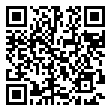• 分享
• 设计
免费设计
• 计算器
装修计算器
• 入驻
合作入驻
• 联系
联系我们
• 置顶
返回顶部